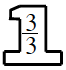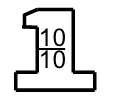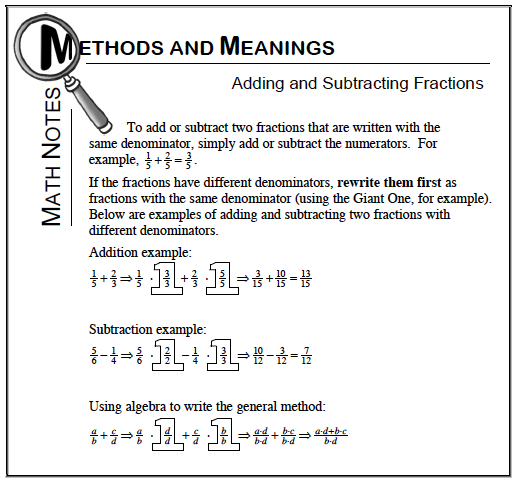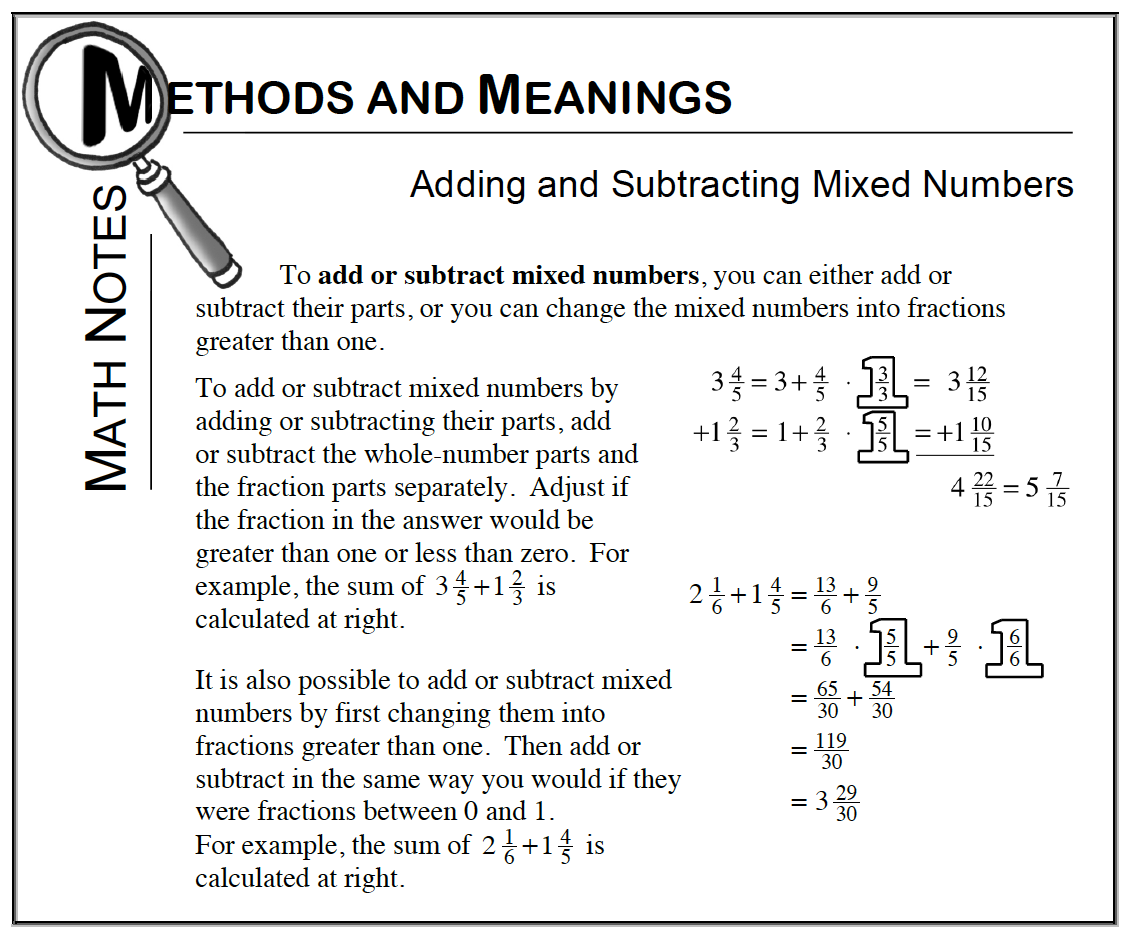### Home > CC1 > Chapter 4 > Lesson 4.1.3 > Problem4-43

4-43.Find each sum or difference without a calculator. Homework Help ✎

1. $\frac { 7 } { 10 } + \frac { 2 } { 3 }$

• For part (a), review the Math Notes box below to find help with adding fractions.

• To add the fractions together, start by finding the least common multiple of 3 and 10. Then use that number as a common denominator. This table lists multiples of 3 and 10.

• $\left. \begin{array} { | c | c | c | c | c | c | c | } \hline 3 & { 6 } & { 9 } & { 12 } & { 15 } & { 18 } & { 21 } & { 24 } & { 27 } & { 30 } & { 33 } \\ \hline 10 & { 20 } & { 30 } & { 40 } & { 50 } & { 60 } & { 70 } & { 80 } & { 90 } & { 100 } & { 110 } \\ \hline \end{array} \right.$

• LCM is 30. Rewrite each fraction to have a denominator of 30 by using Giant Ones, as shown below. Can you add the fractions now?

• $\frac{7}{10}\cdot$$+\ \frac{2}{3}\cdot$$=\frac{21}{30}+\frac{20}{20}$

• $\frac{41}{30}\text{ or }1\frac{11}{30}$1. $0.9−0.04$

Whenever adding or subtracting decimals, be sure to line up the decimal point! It may help to write this problem like this:

$\quad0.90\\-0.04\\\quad\overline { \ ? \ ? ?}$

Also, remember that the decimal point will fall in the same place in your answer.

2. $3 \frac { 1 } { 4 } + 2 \frac { 11 } { 12 }$

Refer to the Math Notes box below.

3. $14 \frac { 1 } { 3 } - 9 \frac { 1 } { 5 }$

• Refer to the Math Notes box below.